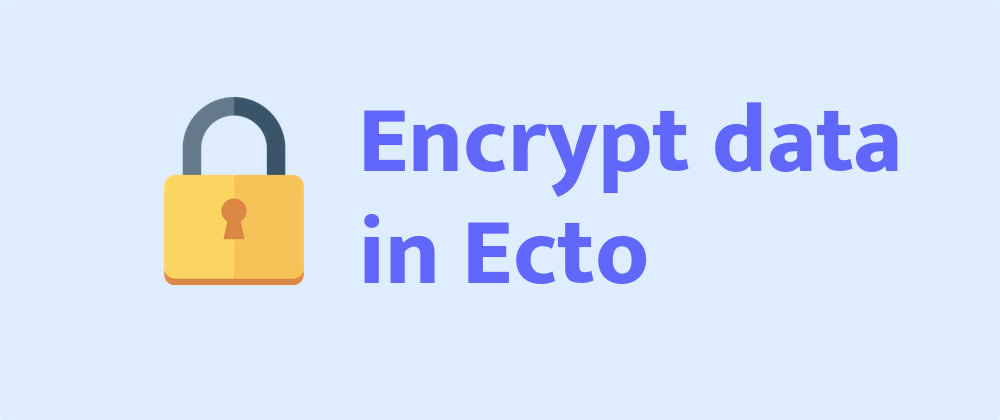# How to encrypt your data with EctoIf your data is encrypted, even if it’s leaked, no one know what is the data. That’s great.

In this post, I’m going to show you how to encrypt data with Ecto. `Ecto` allows developer to define their own types. And we will define a type called `EncryptedText` which encrypts data before persiting to database and decrypts data after loading.

## 1. Define encrypt/decrypt methods

This is a simple version of crypto module:

`````` 1defmodule Crypto do
2  @block_size 16
3
4  def generate_secret do
5    :crypto.strong_rand_bytes(@block_size)
6    |> Base.encode64()
7  end
8
9  def encrypt(plaintext, secret_key) do
10    with {:ok, secret_key} <- decode_key(secret_key) do
11      iv = :crypto.strong_rand_bytes(@block_size)
13      ciphertext = :crypto.crypto_one_time(:aes_128_cbc, secret_key, iv, plaintext, true)
14
15      {:ok, Base.encode64(iv <> ciphertext)}
16    end
17  end
18
19  def decrypt(ciphertext, secret_key) do
20    with {:ok, secret_key} <- decode_key(secret_key),
21         {:ok, <<iv::binary-@block_size, ciphertext::binary>>} <- Base.decode64(ciphertext) do
22      plaintext =
23        :crypto.crypto_one_time(:aes_128_cbc, secret_key, iv, ciphertext, false)
25
26      {:ok, plaintext}
27    else
28      {:error, _} = err -> err
29      _ -> {:error, "Bad encrypted data"}
30    end
31  end
32
34    to_add = block_size - rem(byte_size(data), block_size)
36  end
37
39    to_remove = :binary.last(data)
40    :binary.part(data, 0, byte_size(data) - to_remove)
41  end
42end
``````

Let go through the code

``````1  def generate_secret do
2    :crypto.strong_rand_bytes(@block_size)
3    |> Base.encode64()
4  end
``````

This function generate a 16 bytes secret key and encode base 64 string so you can add it to config.

• `encrypt/2` function
`````` 1 def encrypt(plaintext, secret_key) do
2    # check the key size
3    with {:ok, secret_key} <- decode_key(secret_key) do
4
5      # random initial vector
6      iv = :crypto.strong_rand_bytes(@block_size)
7      # if length of text is not multiple of @block_size
8      # we add more data until it meets condition
10      # encrypt here
11      ciphertext = :crypto.crypto_one_time(:aes_128_cbc, secret_key, iv, plaintext, true)
12
13      {:ok, Base.encode64(iv <> ciphertext)}
14    end
15  end
``````

This is the most important line

``````1ciphertext = :crypto.crypto_one_time(:aes_128_cbc, secret_key, iv, plaintext, true)
``````
• `iv` is initial vector. AES-128 algorithms encrypts data by block of 16 bytes, so we need initial vector to make sure that the output of blocks with same data are different from each other.
• The last parameter is set to `true` to encrypt, set to `false` to decrypt data

And then we encode output to base 64 string. Here we concatenate `iv` and `ciphertext` so that we can extract `iv` to use for decrypting

``````1{:ok, Base.encode64(iv <> ciphertext)}
``````
• `decrypt/2` function
`````` 1def decrypt(ciphertext, secret_key) do
2    # check the key
3    with {:ok, secret_key} <- decode_key(secret_key),
4         {:ok, <<iv::binary-@block_size, ciphertext::binary>>} <- Base.decode64(ciphertext) do
5      plaintext =
6        :crypto.crypto_one_time(:aes_128_cbc, secret_key, iv, ciphertext, false)
8
9      {:ok, plaintext}
10    else
11      {:error, _} = err -> err
12      _ -> {:error, "Bad encrypted data"}
13    end
14  end
``````

We extract `iv` and encrypted data from input

``````1{:ok, <<iv::binary-@block_size, ciphertext::binary>>} <- Base.decode64(ciphertext)
``````

We use pattern matching to extract first 16 byte and assign to `iv` and assign remaining data to `ciphertext`. Then decrypting data

``````1plaintext =
2    :crypto.crypto_one_time(:aes_128_cbc, secret_key, iv, ciphertext, false)
``````

This line is similar to the line which encrypts data, the difference is here we replace `plaintext` by `ciphertext` and last parameter is set to `false`. After data is decrypted, we need to remove padding to get the original data.

## 2. Build `EncryptedText` type

I define a type to store binary data, you can define a `EncryptedMap` to store map data. The most important function are `dump` and `load` where we encrypt before persisting and decrypt after loading.

`````` 1defmodule EncryptedText do
2  use Ecto.Type
3
4  # we store data as string
5  def type, do: :string
6
7  def cast(value) when is_binary(value) do
8    {:ok, value}
9  end
10  def cast(_), do: :error
11
12  def dump(nil), do: nil
13  # encrypt data before persist to database
14  def dump(data) when is_binary(data) do
15    with {:ok, secret_key} <- Application.fetch_env(:myapp, :ecto_secret_key),
16         {:ok, data} <- Crypto.encrypt(data, secret_key) do
17      {:ok, data}
18    else
19      _ -> :error
20    end
21  end
22
23  def dump(_), do: :error
24
26  # decrypt data after loaded from database
27  def load(data) when is_binary(data) do
28    secret_key = Application.fetch_env!(:myapp, :ecto_secret_key)
29    case Crypto.decrypt(data, secret_key) do
30      {:error, _} -> :error
31      ok -> ok
32    end
33  end
34
36
37  def embed_as(_), do: :dump
38end
39
``````

## 3. Use it in your schema

``````1config :myapp, :ecto_secret_key, "your key using Crypto.generate_secret"
``````
``````1schema "users" do
2    field :name, :string
3    ...
4    field :secret, EncryptedText
5    ...
6end
``````

## 4. Conclusion

With Crypto you can implement encrypted field for any type of data you want.

There is an issue when you want to change your secret key, you have to load your data row by row, decrypt and then encrypt with new key and update to database.

I found this article which explains very well about crypto if you are interested https://www.thegreatcodeadventure.com/elixir-encryption-with-erlang-crypto/ Although she uses old crypto API so it will throw some warnings.

I implemented encrypted type for text and map for my company project here if you want to use it:

Github